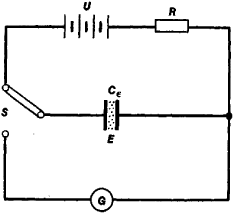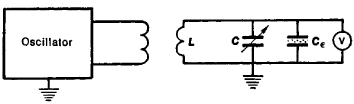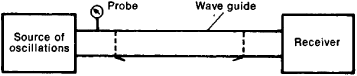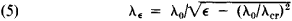# Dielectric Measurements

## Dielectric measurements

Measurements of the dielectric properties of a material, which are characterized by its complex relative permittivity εr. For all materials except ferroelectrics, this quantity does not depend on applied field: the general behavior is linear, and so voltage of any convenient magnitude can be used for measurement. See Ferroelectrics, Permittivity

#### Bridge methods

The most commonly used apparatus for measuring εr is the alternating-current (ac) bridge. These bridges are readily available in the operating range 10–106 Hz, and sometimes outside it; ultralow-frequency bridges can go as low as 10-3 Hz. Most specimen holders for solids are essentially parallel-plate capacitors with the specimen filling all the space between the plates; for liquids, a test cell with cylindrical electrodes is usually employed. The bridges most commonly used are of the Wheatstone type, the most versatile for dielectric measurements being the Schering bridge.

#### Resonance methods

Resonance methods, useful for frequencies greater than 1 MHz, involve the injection of voltage or current by one of several methods into an LC (inductance-capacitance) resonant circuit. Measurements over a range of frequencies may be made by using coils with different inductance values, but ultimately the inductance required becomes impracticably small, and in the range 108-109 Hz reentrant cavities are often used. These are hybrid devices in which the plates holding the specimen still form a lumped capacitor, but the inductance and capacitances are distributed along a coaxial line. At higher frequencies, the wavelength is comparable to the dimensions of the apparatus, and transmission methods in coaxial lines and waveguides must be used.

#### Transmission methods

Coaxial lines are used in the frequency range 300 MHz–3 GHz, and waveguides in the range 3–30 GHz. The transmission characteristics are determined by the complex permittivity of the material filling the line or guide. Many different measurement techniques have been devised, but all derive values of the complex relative permittivity εr from its relationship to the complex propagation factor γ.

In practice, traveling waves are rarely used as the basis of measurement, except for high-loss materials. Usually, reflections from terminations set up standing waves, the amplitude of which in the case of a liquid-filled line can be measured by a suitable probe. The ratios of the field magnitudes at adjacent maxima, and the distance between them, give the information required. See Wavelength

#### Submillimeter measurements

Dielectric measurements are difficult to carry out in the frequency range 30–300 GHz, for which λ0 is in the range 1 cm–1 mm, but for λ0 less than 1 mm, methods related to infrared spectroscopy are used. Broadband continuous spectra result from Fourier transform spectroscopy, which in its simplest form is equivalent to normal infrared spectroscopy, with the specimen in one of the two passive arms of the interferometer, between the beam divider and either the source or the detector. In the more sophisticated dispersive Fourier transform spectroscopy, the specimen is in one of the active arms, that is, between the beam divider and either mirror. Discrete-point spectra also may be obtained by the use of a Mach-Zehnder interferometer and a laser source. By using interferometric techniques, the frequency range can be extended up to about 5 THz. See Infrared spectroscopy, Interferometry, Spectroscopy

#### Time-domain methods

If a constant direct-current (dc) voltage is suddenly applied to a dielectric specimen, in principle the charging current is related through the Fourier integral transformation to the steady-state ac current which would flow if the applied voltage were sinusoidal at any particular frequency. If the dc voltage is suddenly removed, a similar relationship holds between the discharge current and ac current. Thus the variation of complex permittivity with frequency can in principle be derived from a transient signal in the time domain. Because of various limitations, the method is not capable of giving results of an accuracy at all frequencies comparable to those obtainable from a single frequency measurement. Nevertheless, with the aid of computer analysis, the response over a large frequency range can be obtained much more quickly than would be possible by using point-by-point measurement methods.

McGraw-Hill Concise Encyclopedia of Physics. © 2002 by The McGraw-Hill Companies, Inc.
The following article is from The Great Soviet Encyclopedia (1979). It might be outdated or ideologically biased.

## Dielectric Measurements

measurements of quantities that characterize the properties of dielectrics in static and alternating electrical fields. They include measurements of the dielectric constant ∊ in static and alternating fields, dielectric loss, specific electrical conductivity in a constant electrical field, and electric strength.

For solid dielectrics, the measurements often reduce to a determination of the capacitance C of a plane electrical capacitor with the test dielectric placed between its plates. The dielectric constant ∊ is determined from the formula ∊ = (kdlS)C, where d is the thickness of the dielectric specimen, S is the area of its lateral face, and k: is a proportionality factor. For liquids and gases the capacitance of the electrode system is measured in a vacuum (C0) and in the particular substance (C∊), and ∊ is determined from the equation ∊ = Ce/C0.

The methods of measuring capacitance differ for different frequencies of the electrical field. In a constant field and at low frequencies (tenths of a hertz [Hz]), the capacitance is generally determined by measuring the charge or discharge currents of the capacitor with a ballistic galvanometer (Figure 1).Figure 1. Measurement of the dielectric constant with a ballistic galvanometer G

In the frequency range from tenths of a hertz up to 107 Hz it is important to measure, in addition to C, the dielectric loss, which can be expressed by the tangent of the dielectric loss angle, tan δ. The quantities C and tan δ are measured by means of bridge circuits, particularly the Schering bridge.

In the high-frequency range (from 105 to 108 Hz) resonance methods are generally used to measure the capacitance C and the dielectric constant ∊ (Figure 2). An oscillatory circuit that includes a standard capacitor is tuned to resonance and the corresponding value of capacitance C’ is determined. Then a capacitor with the dielectric C is connected in parallel with the standard capacitor and the circuit is again tuned to resonance. In the second case the capacitance C" of the standard capacitor will be smaller. The capacitance of the capacitor that is filled with the dielectric C is found from the formula

(1) C=C1 - C"Figure 2. Measurement of the capacitance C∊ and the dielectric constant by the resonance method. The inductance coil L and the standard capacitor C form a closed circuit that is loosely connected to the AC oscillator.

The various resonance methods are differentiated according to the means of determining tan δ. In the substitution method the dielectric is replaced by an equivalent circuit consisting of a capacitance and a resistance. The resistance R is chosen such that when connected in series or parallel with a standard capacitor C, whose capacitance is taken as equal to the capacitance of the dielectric C, the same resonance current is produced in the circuit as with the specimen dielectric. The circuit detuning method is based on the fact that the width of the circuit’s resonance curve is a function of its quality factor Q, which is related to the tangent of the dielectric loss angle by the equation

(2) tan δ = l/Q

Capacitance and dielectric loss are also determined by means of a Q-meter. In this frequency range it is also possible to use the beat method.

In the superhigh-frequency range (from 108 to 1011 Hz), dielectric measurements are based on the use of cavity resonators and radio wave guides, as well as on the laws governing the propagation of electromagnetic waves in free space. For gaseous dielectrics the resonance frequency ω0and the quality factor Q0 of a cavity resonator are measured (see Figure 3) when a vacuum has been created in it, and the same quantities ω and Q when it is entirely filled with the dielectric. In this case the following relationships exist:

(3) ∊ = (ω0/’ωω)2

(4) tan δ ≈ (1/Q) - (1/Q0)Figure 3. Wave guide apparatus for the measurement of ∊ and τ δ of gases

In the case of liquid and solid dielectrics, much larger changes in the resonance frequency and quality factor are produced if the dielectrics completely fill the resonator. In addition, if the dielectric loss is large, the quality factor of the resonator becomes very small. This invalidates equations (3) and (4). Consequently, partial filling of the resonator with the dielectric, which is frequently in the shape of a disk or rod, is used.

Another method of making dielectric measurements in the superhigh-frequency range involves the establishment of a traveling or a standing wave in a radio wave guide. For a wave guide filled with a dielectric, the wavelength λ1∊iswhere λ0 is the wavelength in free space and λcr is a critical (limiting) wavelength, which is a function of the type of wave and the dimensions of the wave guide’s cross section. The quantity ∊ may be determined from equation (5). When a dielectric is inserted into the wave guide, the wave propagation conditions are changed and the energy of the electromagnetic field is absorbed. This makes possible the determination of tan δ.

There are two main methods of measuring e and tan δ by means of a wave guide. The first is based on the observation of the standing wave pattern in the wave guide, which is loaded with a known resistance. The second is based on an observation of the attenuation of the waves passing through the dielectric. For gases, which have ∊ ≈ 1 and small dielectric losses, ∊ and tan δ are determined by means of the apparatus shown schematically in Figure 3. In the middle section of a wave guide, which is partitioned off by mica windows, a vacuum is created and then the gas is introduced. The wavelength is thus reduced according to equation (5) and the position of the standing wave minimums shifts. Dielectric measurements of liquids and solids having ∊ ≠ 1 are complicated by the reflection of the waves at the air-dielectric interface. Under these conditions the standing wave pattern is observed at the input to the wave guide that is filled with the dielectric by means of a measuring line. In the regions of millimeter, infrared, and light waves either the reflection factor or the index of refraction is measured, together with the absorption coefficient of the dielectric, and from these ∊ and tan δ are found.

The methods of measuring the electrical conductivity cr of dielectrics in a static field are not essentially different from similar methods used for metals and semiconductors. A DC amplifier is used for accurate measurements of very small σ.

Measurements of the electric strength Es are based on measurements of the voltage Vs at which dielectric breakdown occurs:

(6)Es = Vs/d

where d is the distance between the electrodes.

### REFERENCES

Skanavi, G. I. Dielektricheskaia poliarizatsiia i poteri v steklakh i keramicheskikh materialakh c vysokoi dielektricheskoi pronitsaemost’iu. Moscow-Leningrad, 1952.
Karandeev, K. B. Mostovye metody izmerenii. Kiev, 1953.
von Hippel, A. R. Dielektriki i ikh primenenie. Moscow-Leningrad, 1959. (Translated from English.)
Brown, W. Dielektriki. Moscow, 1961. (Translated from English.)
Izmereniia na sverkhvysokikh chastotakh. Edited by V. B. Shteinshleiger. Moscow, 1952. (Translated from English.)

A. N. GUBKIN

Mentioned in ?
References in periodicals archive ?
For this purpose, the dielectric measurements of P(CMEMA-comb-%16St) have been investigated by using the LCR Meter Impedance Analyzer device.
As a matter of fact, the selection of the series of PEAs was also based on the fact that small changes in material properties mainly at molecular level can be easily detected by dielectric measurements. The interest in the dielectric study of aliphatic polyesteramide copolymers becomes necessary due to their increasing applications [5,14-16].
The IoT and related markets for wireless connectivity, coupled by emerging requirements for dielectric measurements in materials science, is driving the need for engineers to add vector network analyzers to their toolboxes.
This paper also describes the selection criteria implemented for heat shrink materials, heat shrink properties, and dielectric measurements in [LN.sub.2] on HTS tapes insulated with heat shrink.
Dielectric measurements in petrophysics and petroleum industry are mostly used to determine the water content.
The dielectric measurements were taken for silver electrode samples using a GW 821 LCR meter over the temperature/frequency range from -75 to 300 [degrees]C and from 20 to 2 MHz, respectively, and under the applied electric field of 20 V/cm.
Physicists describe the experimental growth and characterization of several important dielectric materials using such experimental tools as X-ray diffraction, dielectric measurements, magnetic measurements using a vibrating sample magnetometer, and optical measurements using an ultraviolet-visible spectrometer.
Compact Unfocused Antenna Setup for X-Band Free-Space Dielectric Measurements Based on Line -Network-Network Calibration Method.
To investigate pe effect of fillers in composites of epoxy resin dielectric measurements are performed in pe frequency range from 10-1 Hz to 105 Hz at room temperature as a function of frequency and pickness [11-13].
This is considered a consequence of the low working frequency of the WET sensor (20 MHz), which makes the dielectric measurements more frequency-dependent (Kelleners and Verma 2010).
In practice, we do not have material without losses and cannot perform dielectric measurements without losses.

Site: Follow: Share:
Open / Close